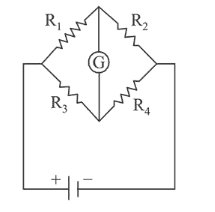# The Wheatstone bridge shown in Fig.Question:

The Wheatstone bridge shown in Fig. here, gets balanced when the carbon resistor used as $R_{1}$ has the colour code (Orange, Red, Brown). The resistors $R_{2}$ and $R_{4}$ are $80 \Omega$ and $40 \Omega$, respectively.

Assuming that the colour code for the carbon resistors gives their accurate values, the colour code for the carbon resistor, used as $R_{3}$, would be:1. (1) Brown, Blue, Brown

2. (2) Brown, Blue, Black

3. (3) Red, Green, Brown

4. (4) Grey, Black, Brown

Correct Option: 1

Solution:

(1) Given, colour code of resistance,

$\mathrm{R}_{1}=$ Orange, Red and Brown

$\therefore \mathrm{R}_{1}=32 \times 10=320$

using balanced wheatstone bridge principle,

$\frac{\mathrm{R}_{1}}{\mathrm{R}_{3}}=\frac{\mathrm{R}}{\mathrm{R}_{4}} \Rightarrow \frac{320}{\mathrm{R}_{3}}=\frac{80}{40}$

$\therefore \mathrm{R}_{3}=160$ i.e. colour code for $\mathrm{R}_{3}$ Brown, Blue and Brown